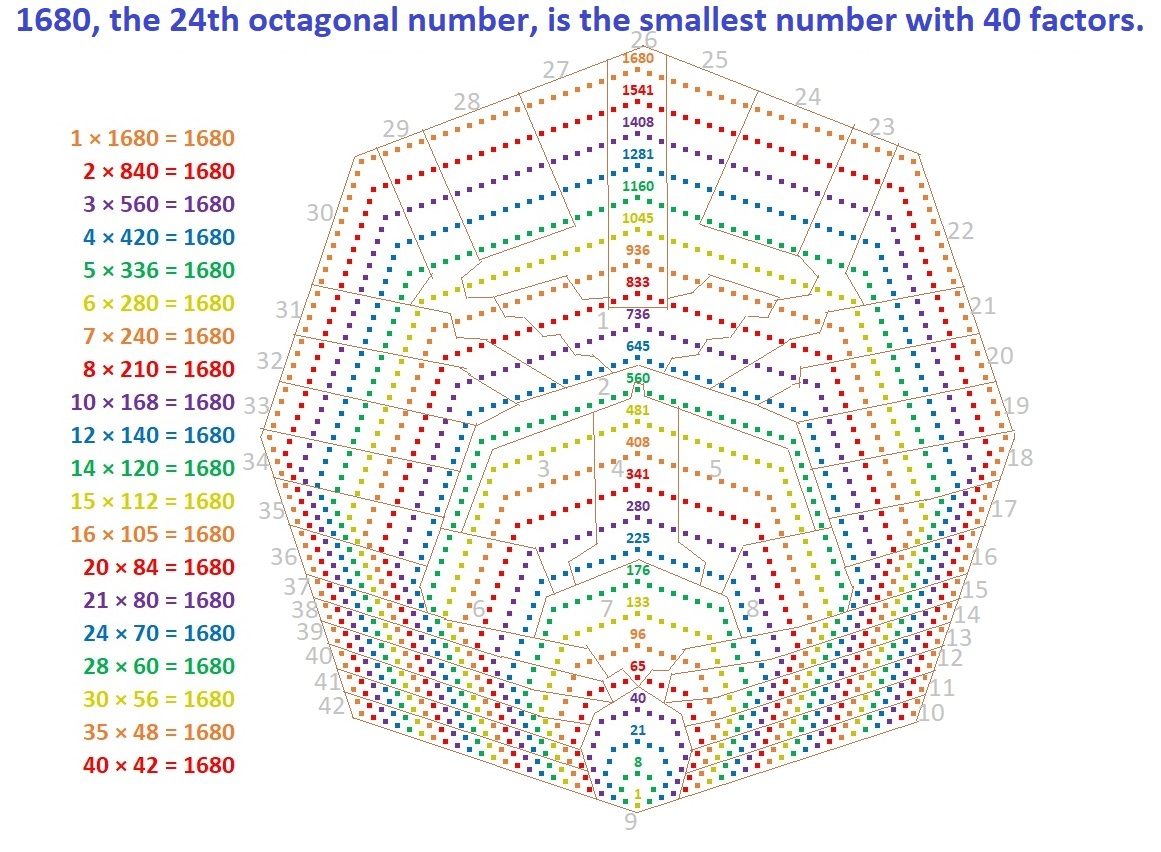# 1680 is a Spectacular Number!

Contents

### Today’s Puzzle:

1680 is the smallest number with at least 40 factors! That fact alone makes 1680 a pretty spectacular number!

Those 40 factors are:
1, 2, 3, 4, 5, 6, 7, 8, 10, 12, 14, 15, 16, 20, 21, 24, 28, 30, 35, 40, 42, 48, 56, 60, 70, 80, 84, 105, 112, 120, 140, 168, 210, 240, 280, 336, 420, 560, 840, and 1680.

Without looking at a list of triangular numbers, determine how many of 1680’s factors are triangular numbers. Then list which factors are triangular numbers. Scroll down to the Puzzle Hints section if needed.

The prime factorization of 1680 is 2⁴ · 3 · 5 · 7.
How many of 1680’s factors are square numbers? What are they?

How many of 1680’s factors are hexagonal numbers? What are they? Scroll down to the Puzzle Hints if you need another puzzle hint.

Each of those questions could be answered by looking at either its list of factors or its prime factorization. The graphic below will help with the next question:
How many of 1680’s factors are octagonal numbers? What are they? The first 24 octagonal numbers are listed along a line in the center of the octagon below.### Puzzle Hints:

Hint for the triangular numbers: The number of triangular numbers is the same as the number of pairs of consecutive numbers listed as factors. For example, 14 and 15 are both factors of 1680, so (14 · 15)/2 = 105 is a triangular number AND it is a factor of 1680.

Hint for the hexagonal numbers: how many of those pairs of consecutive numbers begin with an odd number? For example, 15 is odd and 16 is the next consecutive number. Both of them are factors of 1680, so (15 · 16)/2 = 120 is both a triangular number AND a hexagonal number.

### 1680 Factor Trees:

There are MANY possible factor trees for 1680. The possibilities are more than for any previous number. Since it’s October, I’ll take you into a haunted forest to see three of its trees.If those trees give you the willies, here are three other not-so-scary-looking factor trees:Notice that the prime factors are the same for all of its trees.

### Finding the Factors of 1680:

• You can divide 1680 by 1 because it’s a whole number.
• You can divide it by 2 because it’s even.
• You can divide it by 4 because its last digit is 0, and 8 is even.
• You can divide it by 8 because 80 is divisible by 8, and 6 is even.
• You can divide it by 5 or by 10 because the last digit is 0.
• You can divide it by 3 and/or 7 because these special numbers: 21, 42, 63, 84, 105, 126, 147, 168, and 189 are all divisible by both 3 and 7.
• For the reasons listed above and their prime factorizations, you can also divide 1680 by these composite numbers: 6, 12, 14, 15, 16, 20, 21, 24, 28, 30, 35, 40, and so forth.

Here’s some general factoring information for the number 1680:

• 1680 is a composite number.
• Prime factorization: 1680 = 2 × 2 × 2 × 2 × 3 × 5 × 7, which can be written 1680 = 2⁴ × 3 × 5 × 7.
• 1680 has at least one exponent greater than 1 in its prime factorization so √1680 can be simplified. Taking the factor pair from the factor pair table below with the largest square number factor, we get √1680 = (√16)(√105) = 4√105.
• The exponents in the prime factorization are 4, 1, 1, and 1. Adding one to each exponent and multiplying we get (4 + 1)(1 + 1)(1 + 1) )(1 + 1) = 5 × 2 × 2 × 2 = 40. Therefore 1680 has exactly 40 factors.
• The factors of 1680 are outlined with their factor pair partners in the graphic below.### More about the number 1680:

1680 is the hypotenuse of only one Pythagorean triple:
1008-1344-1680, which is (3-4-5) times 336.

1680 is the difference of two squares in 12 different ways! That’s also more than any previous number, another spectacular fact! Here are those 12 ways:

1.   421² – 419² = 1680,
2.   212² – 208² = 1680,
3.   143²  – 137² = 1680,
4.   109² – 101² = 1680,
5.   89² – 79² = 1680,
6.   76² – 64² = 1680,
7.   67² – 53² = 1680,
8.   52² – 32² = 1680,
9.   47² – 23² = 1680,
10.   44² – 16² = 1680,
11.   43² -13² = 1680, and
12.   41² -1² = 1680.

That last one means we are just one number away from the next perfect square,
41² = 1681. We could predict that as soon as we saw that 40 · 42 = 1680.

The first graphic I made shows 1680 tiny squares arranged into an octagon. I thought it might be fun to use the octagon to demonstrate that 1680 can be divided into 42 groups of 40. It wasn’t always as fun as I thought it would be, but here’s my work:1680, 1681, 1682, 1683, and 1684 are the second smallest set of FIVE consecutive numbers whose square roots can be simplified.(Remarkably, the first number in the smallest set was 1680/2 = 840.) So cool!